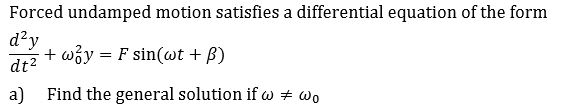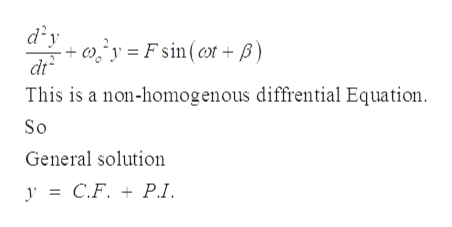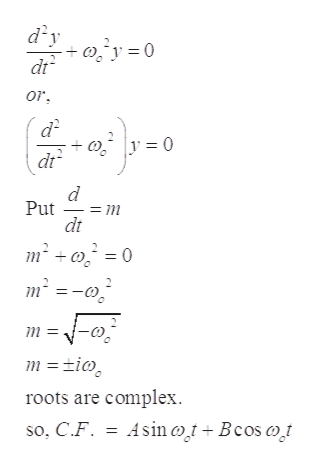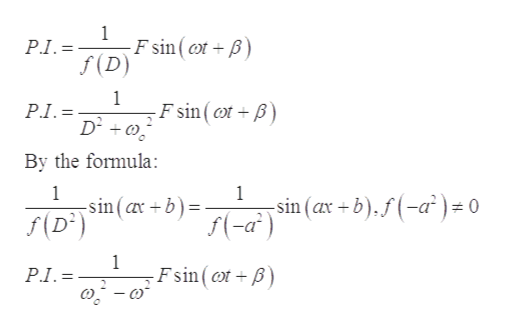# Forced undamped motion satisfies a differential equation of the formd2yby F sin(wt + B)dt2aFind the general solution if=wo

Questionhelp_outlineImage TranscriptioncloseForced undamped motion satisfies a differential equation of the form d2y by F sin(wt + B) dt2 a Find the general solution if = wo fullscreen
check_circleExpert Solution
Step 1

Differential Equation of the undamped forced motionhelp_outlineImage Transcriptionclosedy 'y=Fsin (t+ ) dt This is a non-homogenous diffrential Equation So General solution y C.F. P.1 fullscreen
Step 2

First calculate the C.F. for the equation. Put L.H.S. is equal to the zero.help_outlineImage Transcriptionclosedy dt or |y = 0 dt Put dt m2 0 m tio roots are complex so. C.F . Asinot+Bcos oi fullscreen
Step 3

Now, for calculat...help_outlineImage Transcriptionclose1 F sin (ot B) f (D) P.I.= 1 F sin ( t+ B PI. By the formula: 1 -sin (a b) 1 sin (ax +b).f(-a) f(-a2) 0 f(D 1 Fsin ( t+B P.I.= fullscreen

### Want to see the full answer?

See Solution

#### Want to see this answer and more?

Solutions are written by subject experts who are available 24/7. Questions are typically answered within 1 hour*

See Solution
*Response times may vary by subject and question
Tagged in

### Science